Information on Courses Taught by
Bob Gardner

Here's the information on the courses I am teaching in fall 2023:

 Modern Algebra 1 (MATH 54100) Fall 2023 Complex Analysis 1 (MATH 5510) Fall 2023

For my office hours, click on Homer:Copies of many more syllabi from courses I have taught are available in my syllabus archive.

Copies of many of my class notes are online as given below. I now also have a partial index of the notes available. Some older class notes can be found on my Archives webpage. Additional class notes which are still in preparation are here.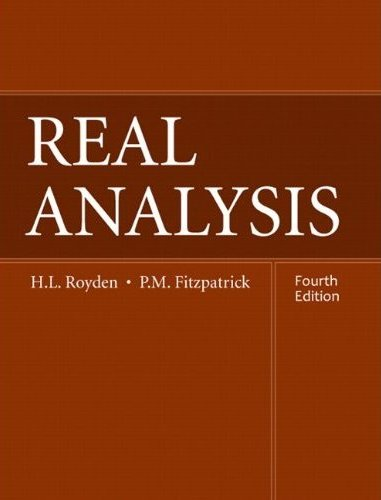Real Analysis Part 1, Part 2, Part 3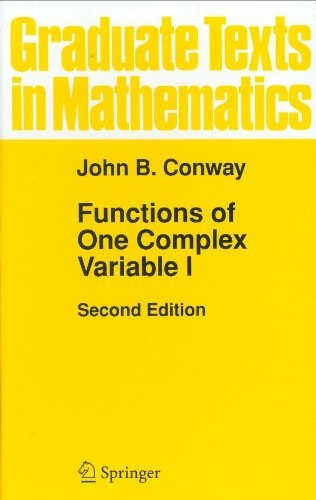Complex Analysis Core, Additional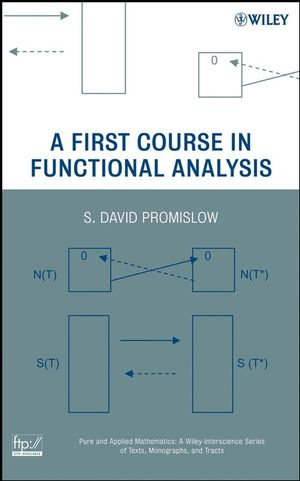Functional Analysis

Graduate Level Algebra, Discrete Math, and Geometry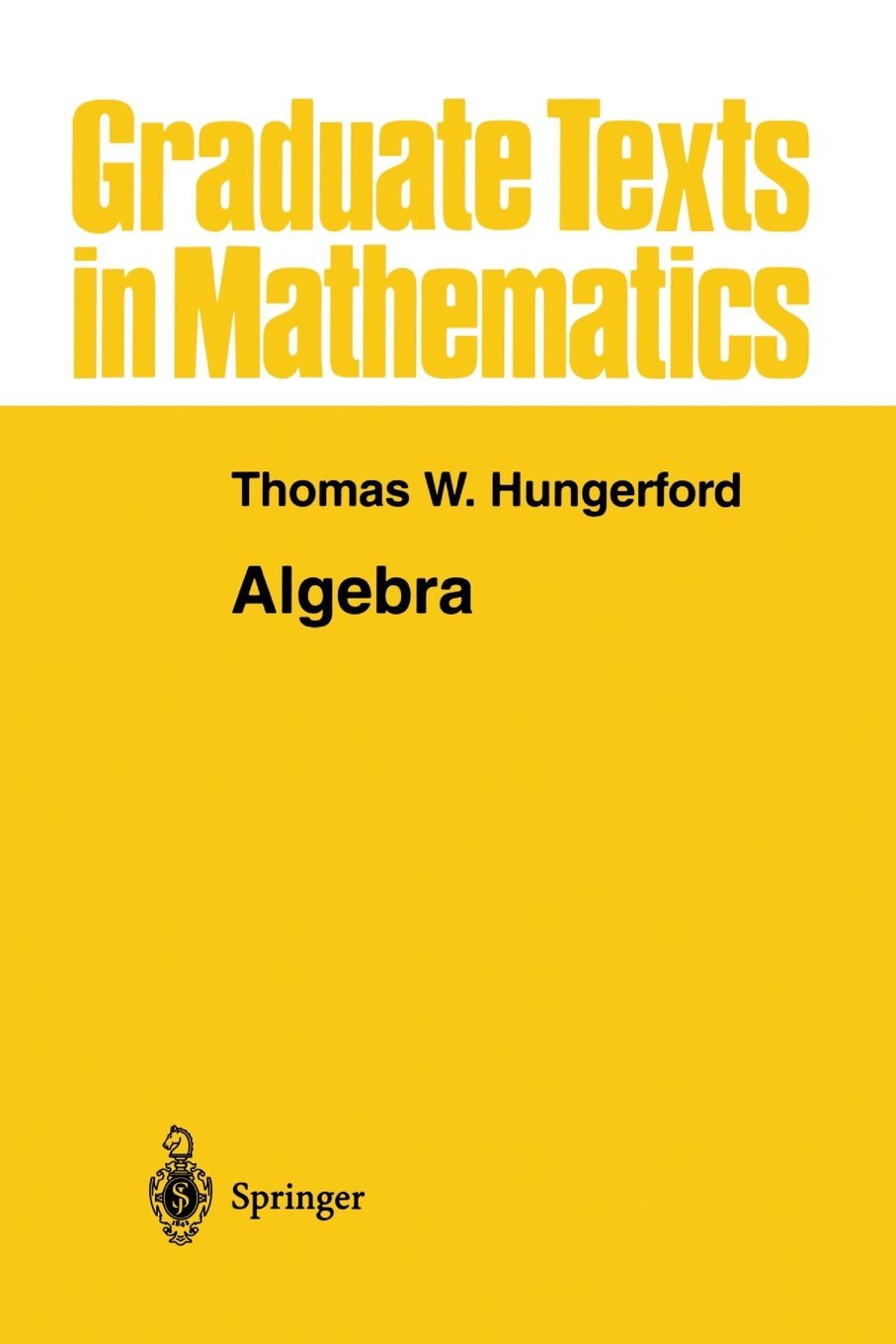Modern Algebra Groups, Rings and Modules, Fields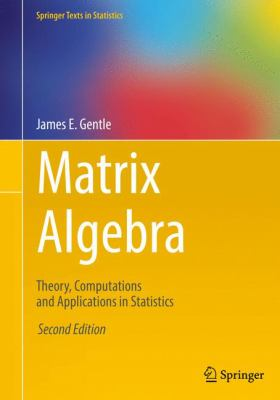Theory of Matrices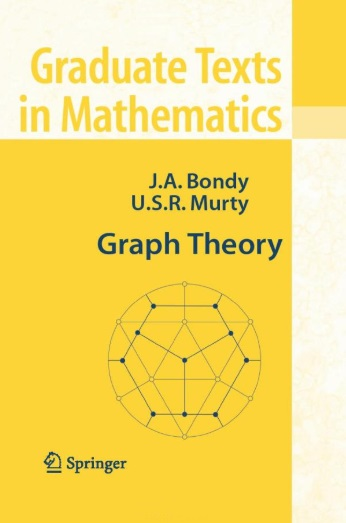Graph Theory 1, Graph Theory 2Mathematical Modeling Using Graph Theory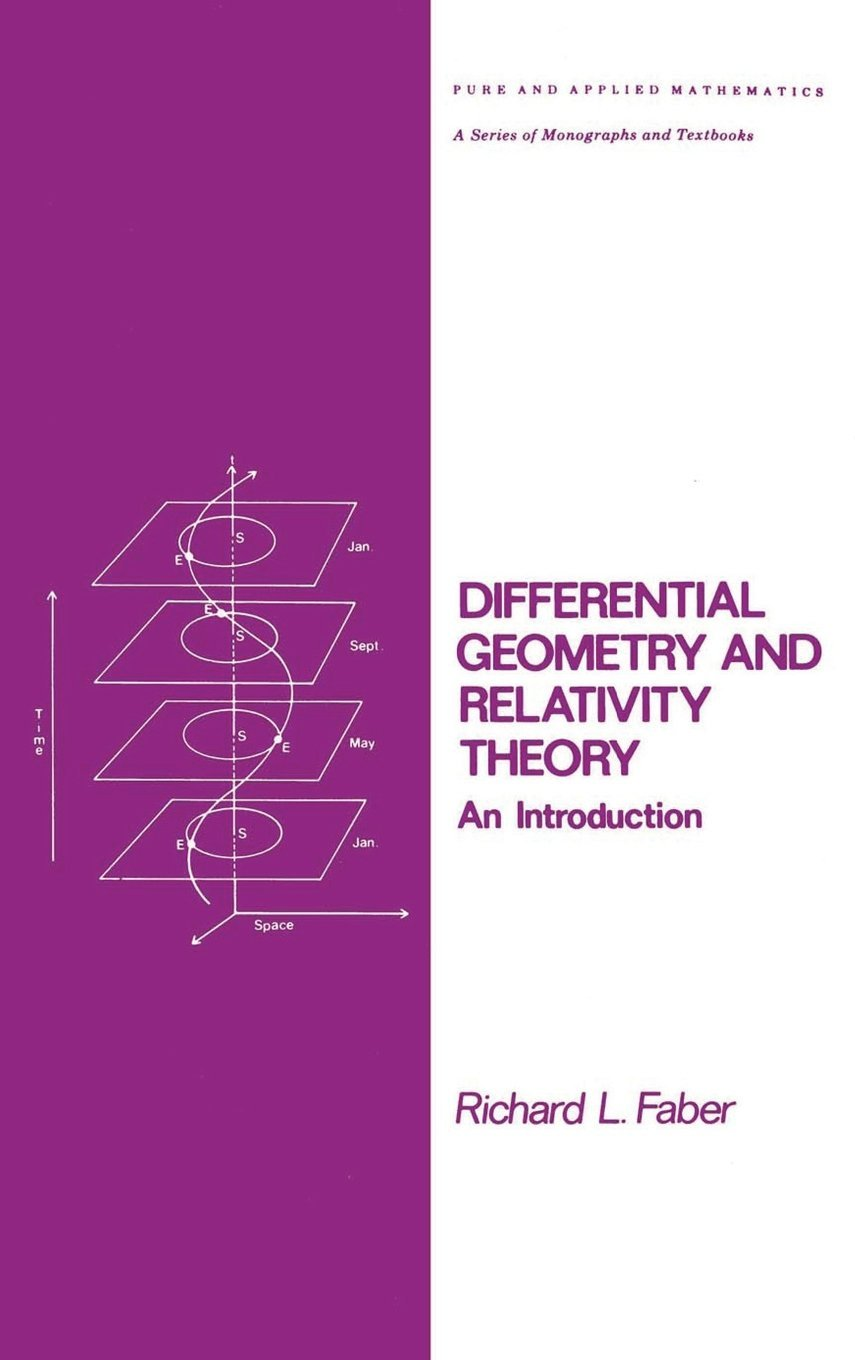Differential Geometry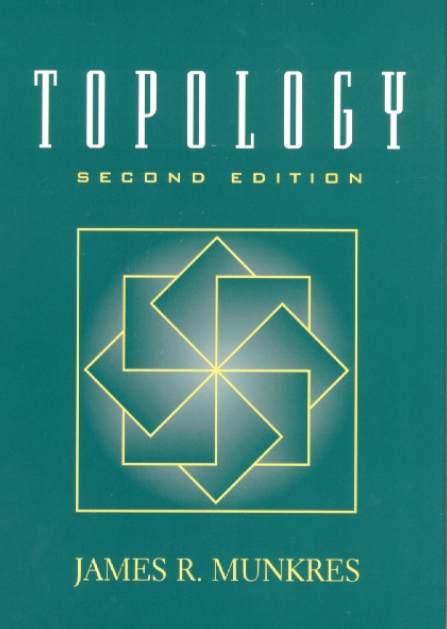General Topology Algebraic Topology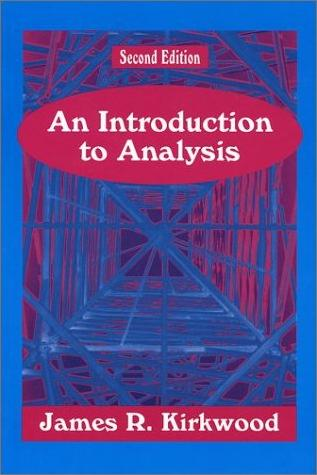Analysis 1 Analysis 2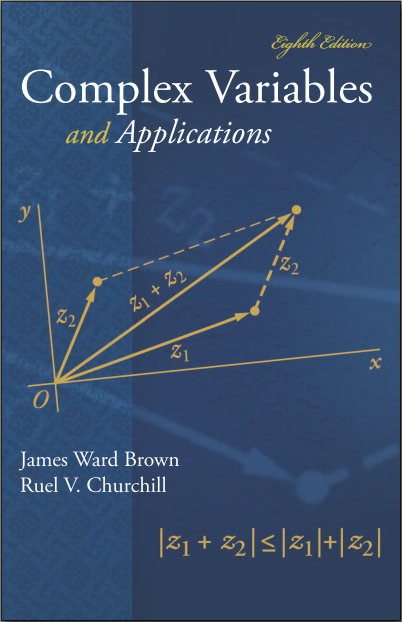Complex Variables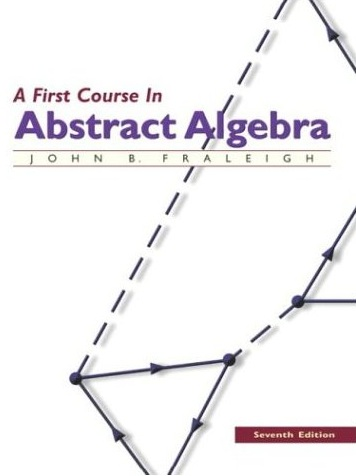Introduction to Modern Algebra Part 1, Part 2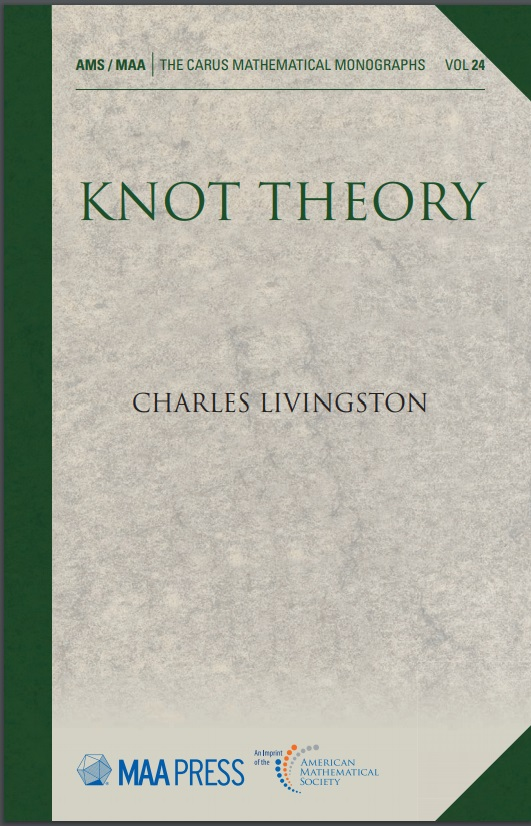Introduction to Knot Theory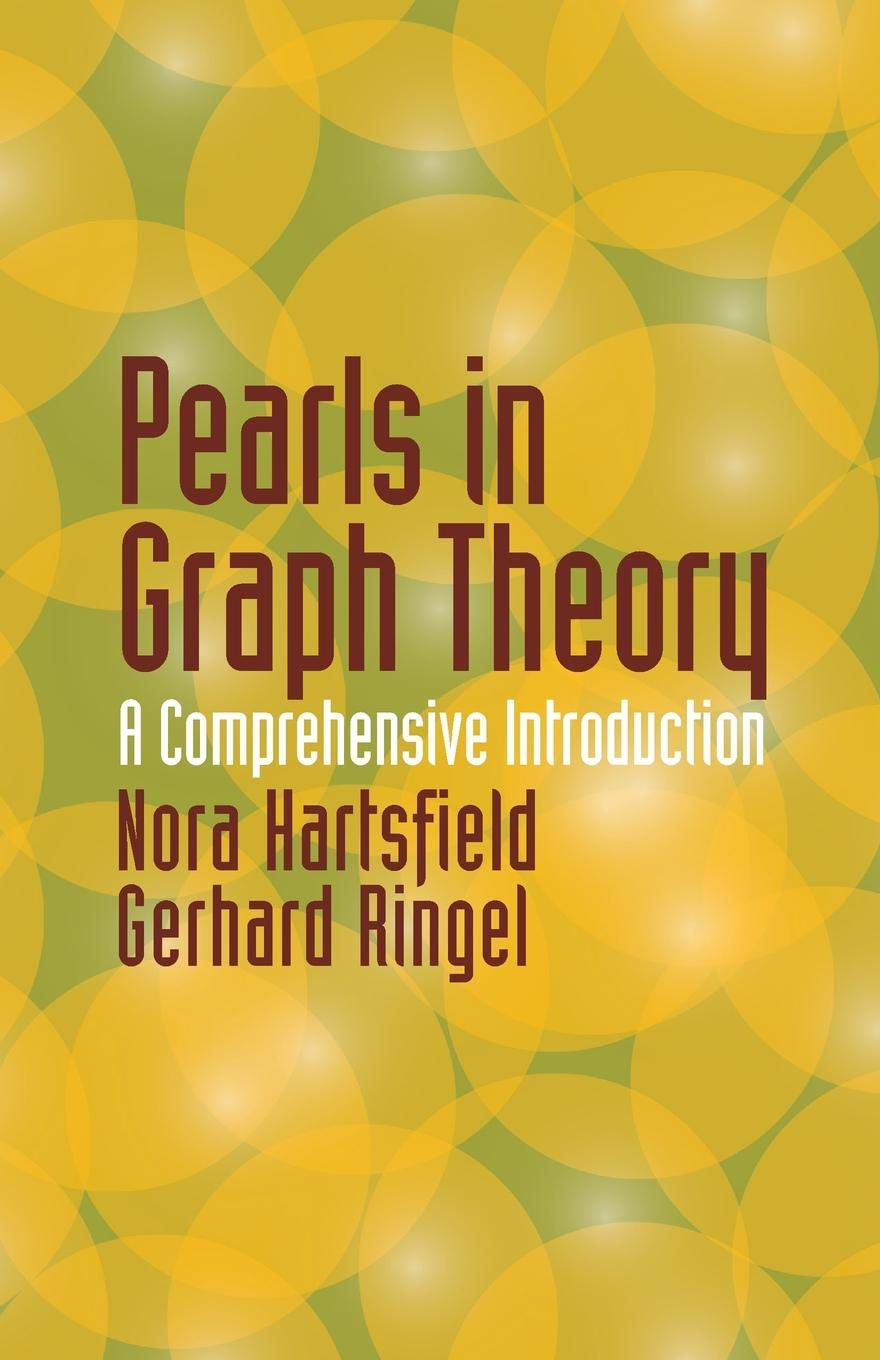Introduction to Graph Theory
Introduction to Modern Geometry
Axiomatic and Transformational Geometry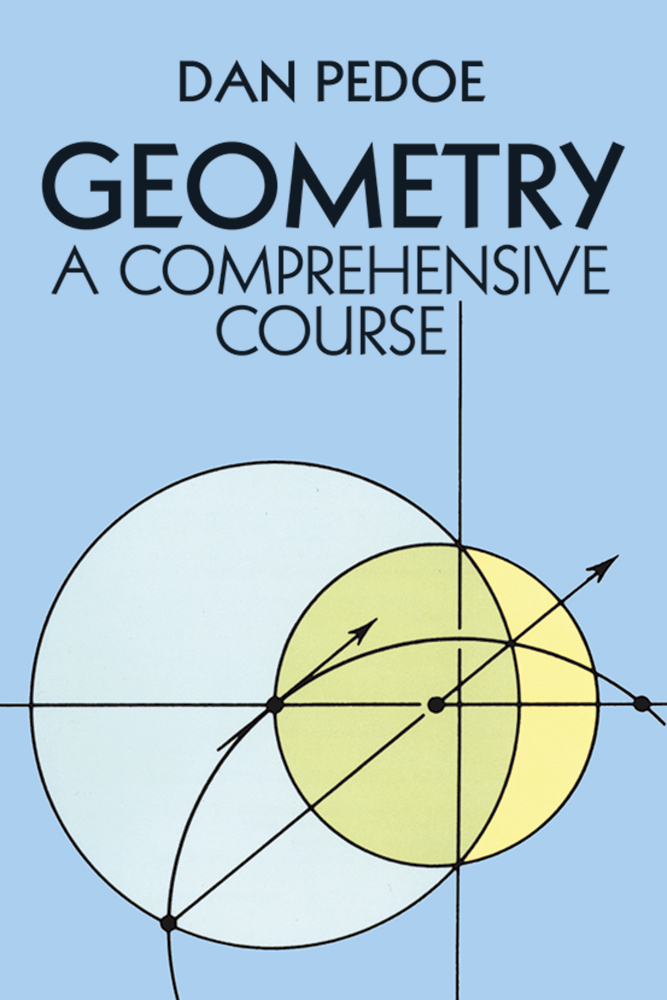Transformational Geometry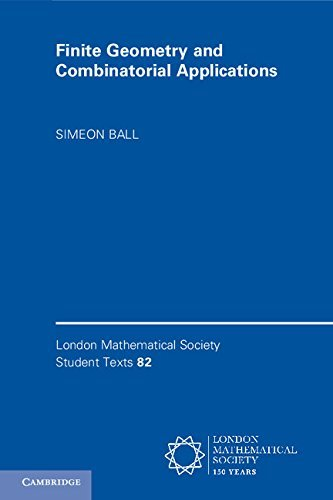Finite Geometry

Some Applied Topics

Freshman, Sophomore, Junior Level

Probability and Statistics

Other

Some solutions to tests which I have given over the past few years are online:

Video lectures prepared for select online classes are available at: